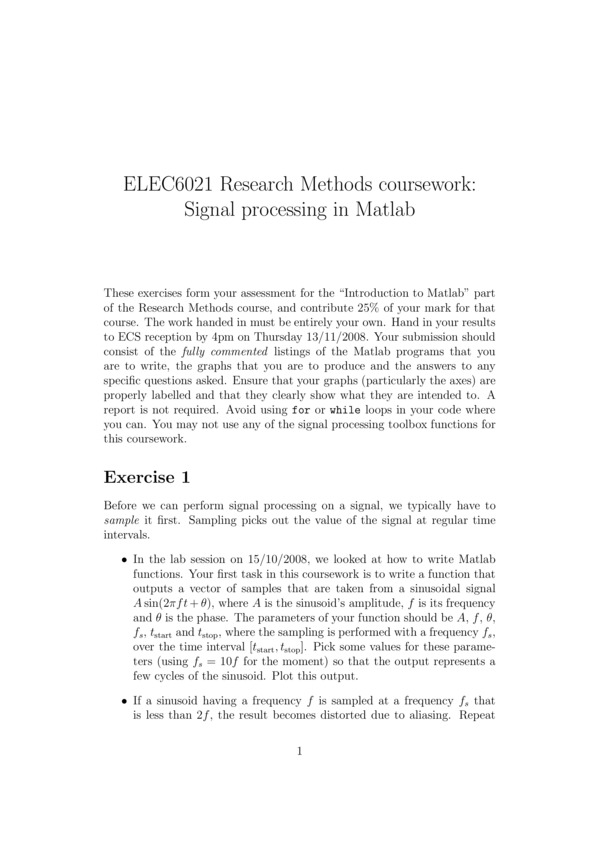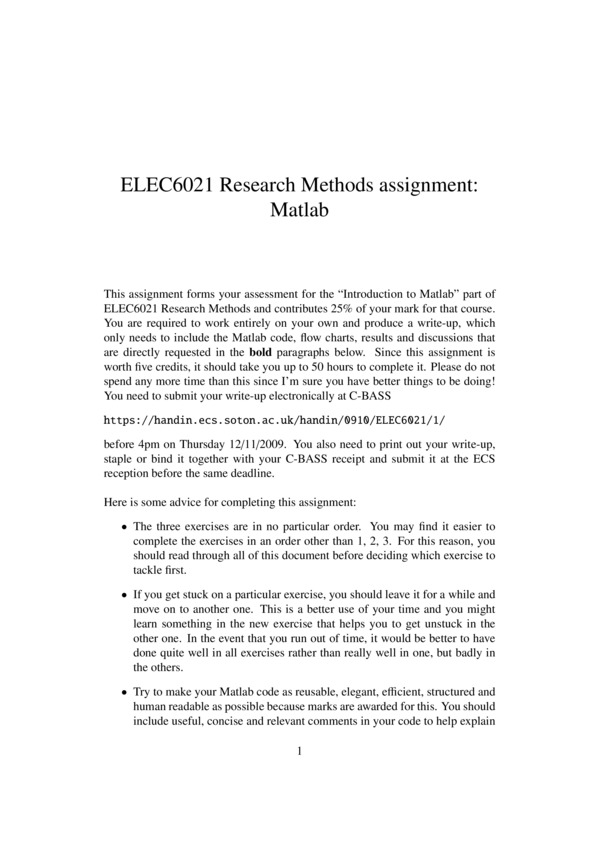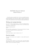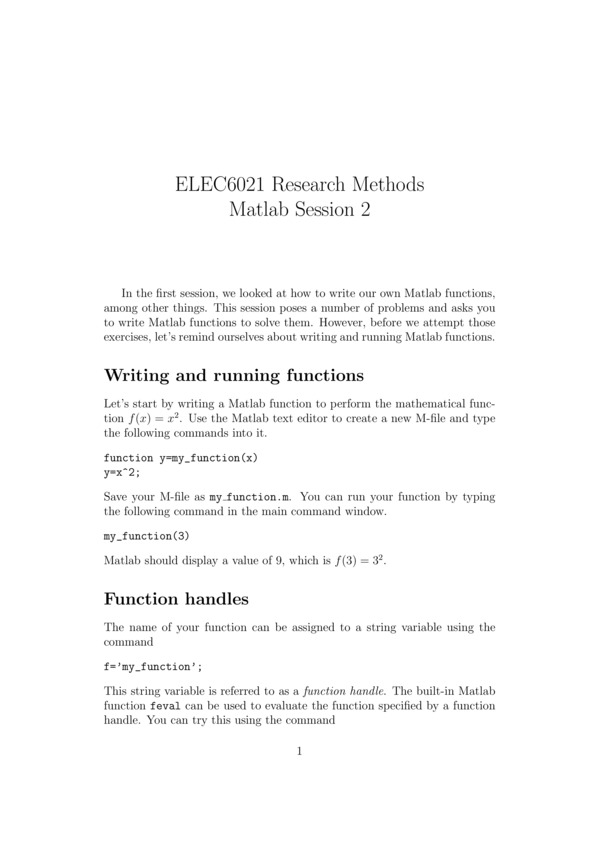# Browse by Tags: matlabUp a level
 Export as ASCII CitationBibTeXDublin CoreEP3 XMLEndNoteExport::Xapian plugin is missing the name parameterHTML CitationJSONMETSMultiline CSVObject IDsOpenURL ContextObjectRDF+N-TriplesRDF+N3RDF+XMLReferReference ManagerZip
Number of items: 11.
1.Preview
Assignment on Matlab
Considers sampling, quantisation, filters and lines of best fit.

Shared with the World by
Prof Rob Maunder
2.Preview
Assignment on Matlab
Considers systems of linear equations, steepest ascent optimisation and Monte Carlo simulation.

Shared with the World by
Prof Rob Maunder
3. Course Notes, Numerical Methods (MATH3018/MATH6111).
Course notes for the Numerical Methods course (joint MATH3018 and MATH6111). Originally by Giampaolo d'Alessandro, modified by Ian Hawke. These contain only minimal examples and are distributed as is; examples are given in the lectures.

Shared with the University by
Dr Ian Hawke
4. Introduction to Matlab - a self-study tutorial
Matlab is a high level language that is very easy to use and very powerful. It comes with a wealth of libraries and toolboxes, that you can use directly, so that you don't need to program low level functions. It enables you to display results very easily on graphs and images. To get started with it, you need to understand how to manipulate and represent data, and how to find information about the available functions. During this self-study tutorial, you will learn: 1- How to start Matlab. 2- How you can find out all the information you need. 3- How to create simple vectors and matrices. 4- What functions are available and how to find them. 5- How to plot graphs of functions. 6- How to write a script. After this (should take about an hour), you will know most of what you need to know about Matlab and should definitely know how to go on learning about it on your own…

Shared with the University by
Ms Debra Morris
5.Preview
Laboratory on Matlab
Considers various basic features of Matlab

Shared with the World by
Prof Rob Maunder
6.Preview
Laboratory on Matlab
Considers function handles, matrix manipulation, 3D plots and programming

Shared with the World by
Prof Rob Maunder
7. Matlab Code for Entropy Coding
Considers Huffman coding and arithmetic coding

Shared with the World by
Prof Rob Maunder
8. Matlab Code for Modulators
Considers Sampling, Pulse Amplitude Modulation, Multiple Access, Quantisation, Pulse Coded Modulation, Manchester Line Coding, Amplitude Modulation, Double SideBand Suppressed Carrier Modulation, Quadrature Amplitude Modulation and M-ary Shift Keying.

Shared with the World by
Prof Rob Maunder
9. Matlab self study tutorial - Graphing
These resources are designed to support students in gaining more confidence with using Matlab. The PDFs provide guidance and information; Objectives: Introduce basic syntax and data preparation for graphing with Matlab by providing some data, examples of code and some background documents. Outcomes: -how to write an m file script -the importance of syntax -how to load files -how to produce simple graphs -where to get help and further examples There are also some data files to provide example data for students to work with in producing Matlab resources.

Shared with the University by
Ms Debra Morris
10. Numerical Methods Worksheets
These worksheets are formative assessment for the Numerical Methods modules MATH3018 and MATH6111 (some material is only covered in MATH3018). Intended to back up both the theory and the coding (in Matlab) side

Shared with the World by
Dr Ian Hawke
11. Numerical Methods lectures for MATH6111 (and MATH3018).
These are the slides used in the joint lectures for MATH3018/MATH6111. They focus on the examples that do not appear in the course notes (see related material). Each lecture comes with example Matlab files that generate the figures used in the lectures.

Shared with the University by
Dr Ian Hawke
This list was generated on Mon Oct 18 16:52:38 2021 UTC.